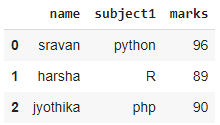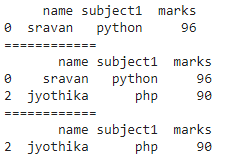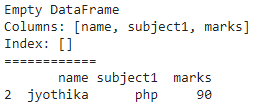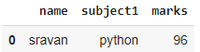Open in App
Not now

# How to Use “NOT IN” Filter in Pandas?

• Last Updated : 22 Nov, 2021

In this article, we will discuss NOT IN filter in pandas, NOT IN is a membership operator used to check whether the data is present in dataframe or not. It will return true if the value is not  present, otherwise false

## Python3

 `# import pandas module``import` `pandas as pd`` ` `# create dataframe``data1 ``=` `pd.DataFrame({``'name'``: [``'sravan'``, ``'harsha'``, ``'jyothika'``],``                      ``'subject1'``: [``'python'``, ``'R'``, ``'php'``],``                      ``'marks'``: [``96``, ``89``, ``90``]}, index``=``[``0``, ``1``, ``2``])`` ` `# display``data1`

Output:sample dataframe

## Method 1: Use NOT IN Filter with One Column

We are using isin() operator to get  the given values in the dataframe and those values are taken from the list, so we are filtering the dataframe one column values which are present in that list.

Syntax: dataframe[~dataframe[column_name].isin(list)]

where

• dataframe is the input dataframe
• column_name is the column that is filtered
• list is the list of values to be removed in that column

## Python3

 `# import pandas module``import` `pandas as pd`` ` `# create dataframe``data1 ``=` `pd.DataFrame({``'name'``: [``'sravan'``, ``'harsha'``, ``'jyothika'``],``                      ``'subject1'``: [``'python'``, ``'R'``, ``'php'``],``                      ``'marks'``: [``96``, ``89``, ``90``]}, index``=``[``0``, ``1``, ``2``])`` ` `# consider a list``list1 ``=` `[``'harsha'``, ``'jyothika'``]`` ` `# filter in name column``print``(data1[~data1[``'name'``].isin(list1)])``print``(``"============"``)`` ` `# consider a list``list2 ``=` `[``'R'``]`` ` ` ` `# filter in name column``print``(data1[~data1[``'subject1'``].isin(list2)])``print``(``"============"``)`` ` `# consider a list``list3 ``=` `[``96``, ``89``]`` ` `# filter in name column``print``(data1[~data1[``'marks'``].isin(list3)])`

Output:NOT IN Filter with One Column

## Method 2: Use NOT IN Filter with Multiple Column

Now we can filter in more than one column by using any() function. This function will check the value that exists in any given column and columns are given in [[]] separated by a comma.

Syntax: dataframe[~dataframe[[columns]].isin(list).any(axis=1)]

## Python3

 `# import pandas module``import` `pandas as pd`` ` `# create dataframe``data1 ``=` `pd.DataFrame({``'name'``: [``'sravan'``, ``'harsha'``, ``'jyothika'``],``                      ``'subject1'``: [``'python'``, ``'R'``, ``'php'``],``                      ``'marks'``: [``96``, ``89``, ``90``]}, index``=``[``0``, ``1``, ``2``])`` ` `# consider a list``list1 ``=` `[``'harsha'``, ``'jyothika'``, ``96``]`` ` `# filter in name and marks column``print``(data1[~data1[[``'name'``, ``'marks'``]].isin(list1).``any``(axis``=``1``)])``print``(``"============"``)`` ` `# consider a list``list2 ``=` `[``'R'``, ``'sravan'``]`` ` `# filter in name and subject1 column``print``(data1[~data1[[``'subject1'``, ``'name'``]].isin(list2).``any``(axis``=``1``)])`

Output:NOT IN Filter with Multiple Column

## Method 3: Use numpy with NOT IN filter

This is similar to the above functionality.

Syntax: dataframe[~numpy.isin(dataframe[‘column’], list)]

## Python3

 `# import pandas module``import` `numpy as np``import` `pandas as pd`` ` `# create dataframe``data1 ``=` `pd.DataFrame({``'name'``: [``'sravan'``, ``'harsha'``, ``'jyothika'``],``                      ``'subject1'``: [``'python'``, ``'R'``, ``'php'``],``                      ``'marks'``: [``96``, ``89``, ``90``]}, index``=``[``0``, ``1``, ``2``])`` ` `# consider a list``list1 ``=` `[``'harsha'``, ``'jyothika'``, ``96``]`` ` `# filter in name column``data1[~np.isin(data1[``'name'``], list1)]`

Output:numpy with NOT IN filter

My Personal Notes arrow_drop_up